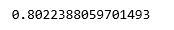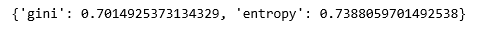# ML | Logistic Regression v/s Decision Tree Classification

Logistic Regression and Decision Tree classification are two of the most popular and basic classification algorithms being used today. None of the algorithms is better than the other and one’s superior performance is often credited to the nature of the data being worked upon.

We can compare the two algorithms on different categories –

Criteria Logistic Regression Decision Tree Classification
Interpretability Less interpretable More interpretable
Decision Boundaries Linear and single decision boundary Bisects the space into smaller spaces
Ease of Decision Making A decision threshold has to be set Automatically handles decision making
Overfitting Not prone to overfitting Prone to overfitting
Robustness to noise Robust to noise Majorly affected by noise
Scalability Requires a large enough training set Can be trained on a small training set

As a simple experiment, we run the two models on the same dataset and compare their performances.

Step 1: Importing the required libraries

 `import` `numpy as np ` `import` `pandas as pd ` `from` `sklearn.model_selection ``import` `train_test_split ` `from` `sklearn.linear_model ``import` `LogisticRegression ` `from` `sklearn.tree ``import` `DecisionTreeClassifier `

Step 2: Reading and cleaning the Dataset

 `cd C:\Users\Dev\Desktop\Kaggle\Sinking Titanic ` `# Changing the working location to the location of the file  ` `df ``=` `pd.read_csv(``'_train.csv'``) ` `y ``=` `df[``'Survived'``] ` ` `  `X ``=` `df.drop(``'Survived'``, axis ``=` `1``) ` `X ``=` `X.drop([``'Name'``, ``'Ticket'``, ``'Cabin'``, ``'Embarked'``], axis ``=` `1``) ` ` `  `X ``=` `X.replace([``'male'``, ``'female'``], [``2``, ``3``]) ` `# Hot-encoding the categorical variables ` ` `  `X.fillna(method ``=``'ffill'``, inplace ``=` `True``) ` `# Handling the missing values `

Step 3: Training and evaluating the Logisitc Regression model

 `X_train, X_test, y_train, y_test ``=` `train_test_split( ` `            ``X, y, test_size ``=` `0.3``, random_state ``=` `0``) ` ` `  `lr ``=` `LogisticRegression() ` `lr.fit(X_train, y_train) ` `print``(lr.score(X_test, y_test)) `Step 4: Training and evaluating the Decision Tree Classifier model

 `criteria ``=` `[``'gini'``, ``'entropy'``] ` `scores ``=` `{} ` ` `  `for` `c ``in` `criteria: ` `    ``dt ``=` `DecisionTreeClassifier(criterion ``=` `c) ` `    ``dt.fit(X_train, y_train) ` `    ``test_score ``=` `dt.score(X_test, y_test) ` `    ``scores ``=` `test_score ` ` `  `print``(scores) `On comparing the scores, we can see that the logistic regression model performed better on the current dataset but this might not be the case always.

My Personal Notes arrow_drop_upCheck out this Author's contributed articles.

If you like GeeksforGeeks and would like to contribute, you can also write an article using contribute.geeksforgeeks.org or mail your article to contribute@geeksforgeeks.org. See your article appearing on the GeeksforGeeks main page and help other Geeks.

Please Improve this article if you find anything incorrect by clicking on the "Improve Article" button below.

Article Tags :
Practice Tags :

1

Please write to us at contribute@geeksforgeeks.org to report any issue with the above content.